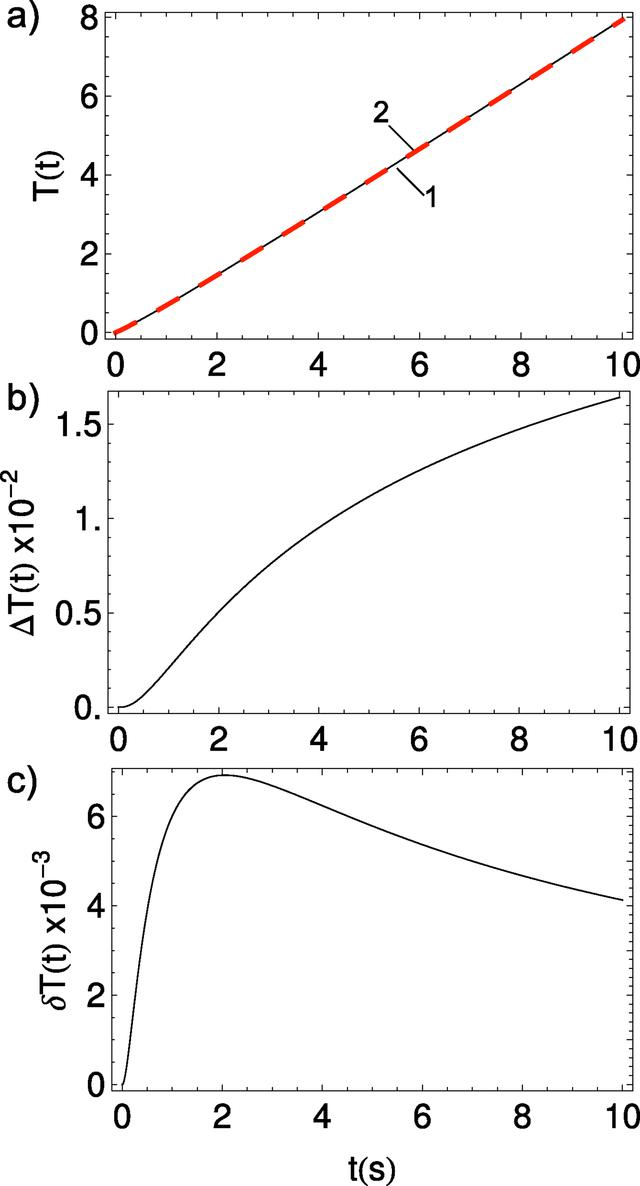disable zoom     view article Figure 6 Optical density A(t) for model 2 (hyperbolic). (a) T(t) integral calculations; the black solid curve (1) is the exact calculation by equation (20), and the red dashed curve (2) shows the approximation by equation (11). (b) Residue ΔT(t). (c) Relative error ΔT(t)/T(t).JOURNAL OFSYNCHROTRONRADIATION
ISSN: 1600-5775
Volume 25| Part 3| May 2018| Pages 833-847